Electron. J. Diff. Equ., Vol. 2012 (2012), No. 107, pp. 1-8.

### Sums of zeros of solutions to second order ODE with non-polynomial coefficients Michael I. Gil'

Abstract:
We consider the equation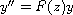(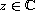) with an entire function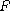satisfying the condition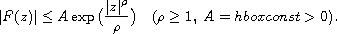Let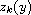,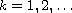be the zeros of a solution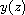to the above equation. Bounds for the sums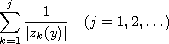are established. Some applications of these bounds are also considered.

Submitted September 8, 2011. Published June 25, 2012.
Math Subject Classifications: 34C10, 34A30.
Key Words: Complex differential equation; zeros of solutions.

Show me the PDF file (216 KB), TEX file, and other files for this article.Michael I. Gil' Department of Mathematics Ben Gurion University of the Negev P.0. Box 653, Beer-Sheva 84105, Israel email: gilmi@bezeqint.net